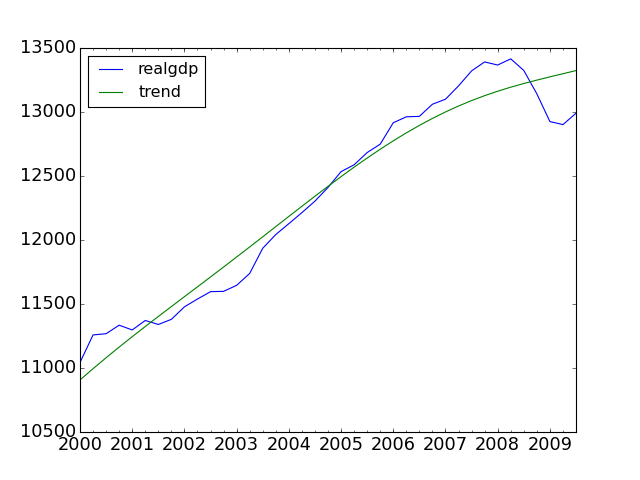# statsmodels.tsa.filters.hp_filter.hpfilter¶

`statsmodels.tsa.filters.hp_filter.``hpfilter`(X, lamb=1600)[source]

Hodrick-Prescott filter

Parameters: X : array-like The 1d ndarray timeseries to filter of length (nobs,) or (nobs,1) lamb : float The Hodrick-Prescott smoothing parameter. A value of 1600 is suggested for quarterly data. Ravn and Uhlig suggest using a value of 6.25 (1600/4**4) for annual data and 129600 (1600*3**4) for monthly data. cycle : array The estimated cycle in the data given lamb. trend : array The estimated trend in the data given lamb.

Notes

The HP filter removes a smooth trend, T, from the data X. by solving

min sum((X[t] - T[t])**2 + lamb*((T[t+1] - T[t]) - (T[t] - T[t-1]))**2)
T t

Here we implemented the HP filter as a ridge-regression rule using scipy.sparse. In this sense, the solution can be written as

T = inv(I - lamb*K’K)X

where I is a nobs x nobs identity matrix, and K is a (nobs-2) x nobs matrix such that

K[i,j] = 1 if i == j or i == j + 2 K[i,j] = -2 if i == j + 1 K[i,j] = 0 otherwise

References

Hodrick, R.J, and E. C. Prescott. 1980. “Postwar U.S. Business Cycles: An
Empricial Investigation.” Carnegie Mellon University discussion paper no. 451.
Ravn, M.O and H. Uhlig. 2002. “Notes On Adjusted the Hodrick-Prescott
Filter for the Frequency of Observations.” The Review of Economics and Statistics, 84(2), 371-80.

Examples

```>>> import statsmodels.api as sm
>>> import pandas as pd
>>> dates = sm.tsa.datetools.dates_from_range('1959Q1', '2009Q3')
>>> index = pd.DatetimeIndex(dates)
>>> dta.set_index(index, inplace=True)
```
```>>> cycle, trend = sm.tsa.filters.hpfilter(dta.realgdp, 1600)
>>> dta["cycle"] = cycle
>>> dta["trend"] = trend
```
```>>> import matplotlib.pyplot as plt
>>> fig, ax = plt.subplots()
>>> dta.ix["2003-03-31":, ["realgdp", "trend"]].plot(ax=ax, fontsize=16);
>>> plt.show()
```